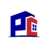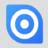sum filtered date in a table`let t := this;`

Hi!

please can you know me how can I sum selected filtered date in a table?

This code doesn't work:

`let bd:= min(Table1.Date)`;

`let ed:= max(Table1.Date);`

`sum((select Table1 where Date >= bd and Date <= ed).Amount)`

`Thank` you!Hi Sal,

`let bd:= min((select Table1).Date);`

`let ed:= max((select Table1).Date);`

`sum((select Table1 where Date >= bd and Date <= ed).Amount)`

Best, JörgHi Jörg,

Thankyou it works!